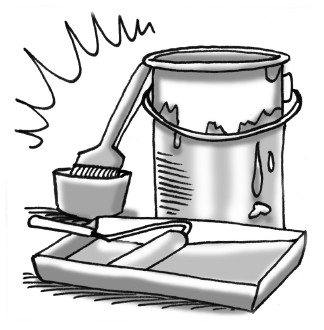### Home > CCG > Chapter 9 > Lesson 9.1.3 > Problem9-38

9-38.

Cindy’s cylindrical paint bucket has a diameter of $12$ inches and a height of $14.5$ inches. If $1$ gallon $≈ 231\text{ in.}^3$, how many gallons does her paint bucket hold?What is the area of the base of the bucket?

Volume of a cylinder = (area of base)(height)

$\pi(6)^2\cdot14.5=522\pi\text{ in.}^3$

$\frac{522\pi \text{ in}^3}{1}\cdot \frac{1\text{ gallon}}{231 \text{ in}^3}\approx 7.1\text{ gallons}$